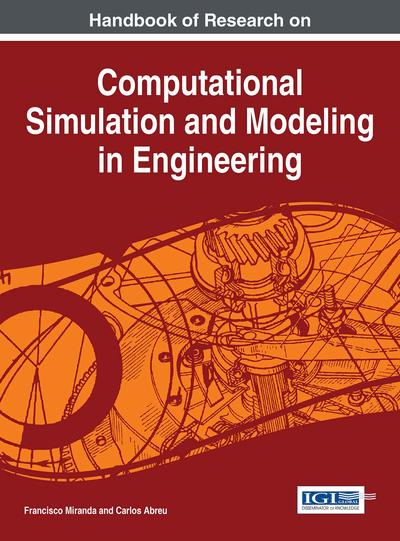# Reliability Analysis of Slope Using MPMR, GRNN and GPR

Dhivya Subburaman (VIT University, India), Jagan J. (VIT University, India), Yıldırım Dalkiliç (Erzincan University, Turkey) and Pijush Samui (National Institute of Technology Patna, India)
DOI: 10.4018/978-1-4666-8823-0.ch007

## Abstract

First Order Second Moment Method (FOSM) is generally for determination of reliability of slope. This article adopts Minimax Probability Machine Regression (MPMR), Generalized Regression Neural Network (GRNN) and Gaussian Process Regression (GPR) for reliability analysis of slope by using FOSM. In this study, an example of soil slope is given regarding how the proposed GPR-based FOSM, MPMR-based FOSM and GRNN-based FOSM analysis can be carried out. GPR, GRNN and MPMR have been used as regression techniques. A comparative study has been carried out between the developed GPR, MPMR and GRNN models. The results show that MPMR gives better performance than the other models.
Chapter Preview
Top

## Introduction

The determination of reliability of slope is a challenging task in civil engineering. Researchers use First Order Second Moment Method (FOSM) has been used for reliability analysis of slope by many researchers (Wu and Kraft, 1970; Cornell, 1971; Alonso, 1976; Tang et al., 1976; Vanmarcke, 1977; Husein et al., 2000). However, FOSM is a time consuming method for implicit performance function. Implicit performance function is common for Bishop (1955) method of slope stability analysis. Bishop (1955) used the method of slices in obtaining stability of slopes. In order to solve the problem, Bishop assumed that the resultant of interslice forces acts in the horizontal direction. This method is being used very widely in the literature. The results obtained from this method compares very closely with the more rigorous approaches like the finite element method. Researchers try to overcome this problem by using different approaches such as Response Surface Method (Wong, 1985; Faravelli, 1989; Bucher and Bourgund, 1990; Rajashekhar and Ellingwood, 1993; Kim and Na,1997; Guan and Melchers, 2001; Kaymaz and McMahon, 2004; Nguyen et al., 2009; Roussouly et al., 2013; Jiang et al., 2014b), multi-plane surfaces method, and multi-tangent-plane surface (Guan and Melchers, 1997). But, these approaches are only appropriate for nonlinear concave or convex limit state surface. Researchers use Artificial Neural Networks (ANNs) to slope the problem of implicit performance (Hurtado and Alvarez, 2001; Gomes and Awruch, 2004; Deng Jian et al., 2005; Deng Jian, 2006; Elhewy et al., 2006; Cardoso et al., 2008). However, ANN suffers from the different limitations such as black box approach, low generalization capability, overtraining problem, arriving at local minima, etc (Park and Rilett, 1999; Kecman, 2001).

## Key Terms in this Chapter

Generalized Regression Neural Network: A generalized regression neural network is often used for function approximation. It has a radial basis layer and a special linear layer.

First Order Second Moment Method: First Order Second Moment Method considers the parameter as uncertain variables. In this, the mean and covariance are determined by using moment values of the parameters. Then the model was developed based on constraint state formulation.

Gaussian Process Regression: Gaussian process regression is an infinite-dimensional generalization of the multivariate normal distribution.

Minimax Probability Machine Regression: It is defined as the process of maximizing the minimum probability of regression model for all possible distribution with known mean and covariance matrix.

Reliability: Consistency and validity of test outcomes determined through statistical methods after repeated trials.

Slope Stability: The resistance of an inclined surface of the soil to failure by sliding or collapsing.

## Complete Chapter List

Search this Book:
Reset